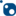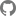Euclid is a .NET library for calculating position and rotation in three-dimensional Euclidean spaces. It depends on .NET Standard 2.0.

## Get started

Maroontress.Euclid is available as theNuGet package.

## Examples

### Position and XYZ tuple

You can use the `Position` class and its extension methods to manipulate coordinates in three-dimensional space. Instances of the `Position` class are immutable objects, so the operations that return a `Position` object create a new instance as follows:

``````// 'p' represents (x, y, z) = (1, 2, 3).
var p = new Position(1, 2, 3);
// 'q' represents (x, y, z) = (4, 5, 6).
var q = new Position(4, 5, 6);
var r = p.Add(q);

// p == (1, 2, 3)
// q == (4, 5, 6)
// r == (5, 7, 9)
``````

You can also use XYZ tuples with extension methods. Note that an XYZ tuple (structure) is not immutable. The extension methods do not change its value but return a new instance, as do the methods of the `Position` class as follows:

``````// 'p' represents (x, y, z) = (1, 2, 3).
var p = (1.0f, 2.0f, 3.0f);
// 'q' represents (x, y, z) = (4, 5, 6).
var q = (4.0f, 5.0f, 6.0f);
var r = p.Add(q);

// p == (1, 2, 3)
// q == (4, 5, 6)
// r == (5, 7, 9)
``````

### Rotation

The `Matrix33` class can be used as a rotation matrix to handle rotation in three-dimensional space. Note that the `Matrix33` class itself can represent 3 × 3 square matrices.

You can create an instance with three column vectors as follows:

``````var x = new Position(1, 1, 1).Normalize();
var y = new Position(-1, 0, 1).Normalize();
var z = x.CrossProduct(y);

// R = (X Y Z)
var r = new Matrix33(x, y, z);
``````

Each column vector is a projection of a unit vector along the X, Y, and Z axes.

You can project positions with the rotation matrix as follows:

``````var m = new Matrix33(x, y, z);
var p = new Position(1, 1, 1);
var q = m.Map(p);
``````

Instances of the `Matrix33` class are immutable objects, so the operations that return objects of the `Matrix33` class create new instances as follows:

``````var t = new Matrix33(x1, y1, z1);
var u = new Matrix33(x2, y2, z2);

// V = TU
var v = t.Mul(u);
``````

### Posture

Each instance of `Posture` class contains a pair of a position and a rotation matrix, which represents translation and rotation relative to the origin.

You can create a `Posture` instance with `Position` and `Matrix33` objects as follows:

``````var p = new Position(...);
var r = new Matrix33(...);
var pose = new Posture(p, r);
``````

The `Posture` object allows you to transform positions from a parent coordinate system to the child coordinate system, or vice versa.

### Coordinate system

The instance of `CoordinateSystem` class represents a singly-linked list where each node contains a `Posture` object. The link of each coordinate system stands for its parent.

The `World` object is the head node of the list. Its parent is itself.

``````var sunPosture = new Posture(...);
var earthPosture = new Posture(...);
var moonPosture = new Posture(...);

var world = CoordinateSystem.World;
var sun = world.NewChild(sunPosture);
var earth = sun.NewChild(earthPosture);
var moon = earth.NewChild(moonPosture);
``````

The `CoordinateSystem` object allows you to transform positions from the world coordinate system to the local coordinate system, or vice versa.

## How to contribute

Please send us pull requests or issues from theGitHub repository.